# Critical function

(diff) ← Older revision | Latest revision (diff) | Newer revision → (diff)
A statistic for which the values are the conditional probabilities of the deviations from the hypothesis being tested, given the value of an observed result. Letbe a random variable with values in a sample space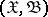, the distribution of which belongs to a family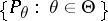, and suppose one is testing the hypothesis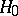:, against the alternative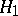:. Let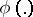be a measurable function on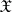such that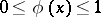for all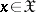. If the hypothesis is being tested by a randomized test, according to whichis rejected with probability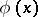if the experiment reveals that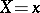, and accepted with probability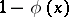, thenis called the critical function of the test. In setting up a non-randomized test, one chooses the critical function in such a way that it assumes only two values, 0 and 1. Hence it is the characteristic function of a certain set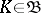, called the critical region of the test: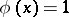if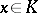,if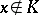.